PPWIZARD is a free preprocessor for HTML, REXX, Visual Basic or any text files.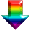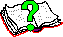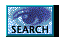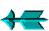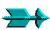\ -> Rexx -> Standard Rexx Routines

 SUMMARY OF REXX "BUILT IN" ROUTINES

You can get a rexx manuals (for regina) from http://www.lightlink.com/hessling/, there are HTML and PDF versions available.

Note that some of the routines listed below may not be available in the rexx version you are using (neither does it list all functions):

```ABBREV(information,info[,length])  - check for valid abbreviations
ABS(number)                        - return the absolute value
B2D(binary)
B2X(binary)
D2B(decimal)
C2X(string)
C2D(string[,n])
D2C(decimal[,n])                   - convert between data formats
D2X(decimal[,n])
X2B(hex)
X2C(hex)
X2D(hex)
CENTER(s,n[,pad])                  - centre a string in a field

COPIES(s,n)                        - replicate a string
DATATYPE(string[,type])            - test datatype. Type can be:
A (alphanumeric) B (bits) L (lowercase)
M (mixed case) N (number) S (symbol chars)
U (upper case) W (whole number) X (hex)

DATE([format])                     - get date. Format can be:
B (base date - days since 1/1/1 AD)
C (days in century) D (days in year)
E (European) J (Julian) M (month name)
N (normal: dd Mon yyyy) O (ordered)
S (sorted) U (USA) W (day of week)

FORMAT(number [,[before] [,[after] [,[expp] [,expt]]]] )
- format a number as specified
FUZZ()                             - NUMERIC FUZZ setting
INSERT(new,target[,[n][,[length][,pad]]])  - insert new string into target
JUSTIFY(s,n[,pad])                 - justify text to given width
LASTPOS(needle,haystack[,start])   - find last occurrence of a string
LEFT(string,num[,pad])             - return an initial substring
LENGTH(string)                     - find the length of a string
MAX(number[,number...])            - find the maximum of a set
MIN(number[,number...])            - find the minimum of a set
OVERLAY(new,target[,[n][,[length][,pad]]])  - overlay new string on to target
POS(needle,haystack[,start])       - find the first occurance of a string
RANDOM([min][,[max][,seed]])       - return a random number
REVERSE(string)                    - find the reverse of a string
RIGHT(string,num[,pad])            - return a final substring
SPACE(s[,[n][,pad]])               - evenly space words in a sentence
SUBWORD(string,n[,k])              - return a substring of words
SYMBOL(name)                       - test to see if a symbol is defined
TIME([format])                     - get the time. Format can be:
C (civil time) N (normal) L (long)
H (hours since midnight)
M (minutes since midnight)
S (seconds since midnight) E (elapsed time)
R (elapsed time then reset)

- translate characters using given tables
TRUNC(number[,n])                  - truncate floating point number
VALUE(s[,[newvalue][,selector]])   - get or set value of a symbol

VERIFY(string,reference[,[option][,start]])
- verify string for valid characters
WORD(string,n)                     - return a word from a string
WORDINDEX(string,n)                - return the position of a word in a string
WORDLENGTH(string,n)               - return the length of a word in a string
WORDPOS(phrase,string[,start])     - find a phrase in a string
WORDS(string)                      - return the number of words in a string
XRANGE([a[,b]])                    - return a range of characters
```

Some rexx interpreters support functions which are not ANSI standard, others won't support all standard ANSI routines. If you care about operating system portability you will be careful about which routines you use. PPWIZARD has its own routines which may be be used, these should be used in preference to "standard" rexx ones as they will work in all operating systems that PPWIZARD supports.

Saturday May 28 2022 at 2:55pm